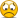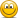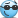# Tuckman, Chapter 6: Empirical Approaches to Risk Metrics and Hedging

#### David Harper CFA FRM

##### David Harper CFA FRM
Staff member
Subscriber
Hi @cfa2015 Your right, it's true that the negative doesn't follow through (it doesn't in the source Tuckman). It's negative to indicate/reflect the hedge: the underlying exposure is a $100.0 short position position in the "nominal" Treasury bonds and the hedge is a long (purchase) of TIPS which is solved-for to be$73.33. So the negative just reflects that the hedge will be short against a long or vice-versa. We did follow Tuckman. If the math were pure, I think the "F Nominal input" should be -100 (i.e., short) such that the output would be +77.7773 (i.e., long). I hope that clarifies, thanks!

#### jbejarjimenez

##### New Member
Hi, It may seem a pretty basic question, but I have realized that many people have the same doubt. Why we use a regression hedge to estimate the proper hedging? Assuming that you discount both instruments with the same rate (eg: spot rates) the DV01 already accounts for the difference between TIPS instruments and standard T-Bonds.

#### David Harper CFA FRM

##### David Harper CFA FRM
Staff member
Subscriber
HI @jbejarjimenez When you hedge per F(hedge) = -F(exposure)*DV01(exposure)/DV01(hedge), you are equalizing (neutralizng) the DVO1 (aka, dollar duration/10000). When the dollar duration is equal for both, a yield shock of xΔ% to both implies that the dollar change to both is equal, and the hedge works. So the premise in neutralizing DV01 is that yield shocks are the same to both positions. But a regression attempts to correct for this. For example, in Tuckman's example in the text of Chapter 6, the hedge instrument's (TIPS) yield, on average, shocks a little less than the yield shock to the exposure (T-bond), so the regression-based approach hedges with slightly more TIPS to correct for this tendency. In the unadjusted 1:1 hedge, a yield shock of xΔ% to yield of the exposure would imply a slightly insufficient dollar-change to the hedge instrument, but the adjusted amount ensures that the dollar change of the hedge is equal. In this way, an unequal (on average) yield shock is translated into an (expected) equal change to dollar amounts. Thanks,

#### jbejarjimenez

##### New Member
Hi David, thank you very much for your detailed answer, but I still don't completely get the point. I'm going to try to explain better myself giving an example:
Imagine a trader goes short $100m of Treasury bonds which have a DV01 of 0,025, meaning that when the spot treasury curve (not the yield implied in the security as far as I know, correct me if I'm wrong) drops 1bp the Treasury bond goes up by$0,025m. Now you have a TIPs which has a DV01 of 0,015 that you are thinking about using as a hedge instrument, this DV01 of 0,015 already tells you that the shock is less on TIPS as you say, and therefore no regression is needed.
Please correct me if I'm wrong, I know that this is a pice of cake but I cannot move forward without having a complete understanding of why we use a regression hedge.

#### David Harper CFA FRM

##### David Harper CFA FRM
Staff member
Subscriber
Hi @jbejarjimenez no worries, i don't think it's a piece of cake for anybody. In the un-adjusted approach, you buy whatever amount of the hedge instrument to set equal (per a simple re-arrangement): F(hedge)*DV01(hedge) = F(exposure)*DV01(exposure). That's really what I mean by equalizing/neutralizing the dollar duration. Using your example, when we use this un-adjusted approach, when the nominal spot treasury curve drops 1 bp, the value of the Treasury bond position goes up by 0.025 /100 * F(T-bond) position dollars; if at the same time, the real spot treasury curve also drops by exactly 1 bp (this is the implicit assumption ...) then the TIPS position will drop by exactly the same dollar amount; i.e., not DVO1, but 0.015 /100 * Face(TIPS). This is by definition of the neutralizing formula used. On the other hand, the adjustment incorporates the regression-informed idea that the real spot treasury curve will drop simultaneously by less than < 1 bp.

I followed you with the assumption of a one point drop in the spot (aka, zero) rate curve because it's generally a true approximation to the actual assumption, which is: these are single-factor yield-based DV01, as in "yield to maturity." So it's actually an assumption of matching drops in the yield-to-maturity. However, a one point drop in the yield does approximate a parallel shift in the zero rate curve, so it's fine to say "parallel drop in the spot rate curve." I hope that clarifies!

Last edited:

#### jbejarjimenez

##### New Member
Hi David, thank you very much you have clarified that for me perfectly. Great explanation#### Dips

##### New Member
Hello, #myfirstpost. Can we go a step back and look at the p&l equation?
I don't understand why both the nominal and real terms are negative.
If I have sold something (treasuries/nominal) to buy something else (TIPS/inflation/real) then surely my payoff will be
+ what I am long - what I am short.
I'm completely missing something.

#### David Harper CFA FRM

##### David Harper CFA FRM
Staff member
Subscriber
Hi @Dips Welcome! Your #firstpost coincides with the first workday of our new upgraded forum, yipee!Yes, that's yet another confusing element. It's really because the DV01 is denoted like duration: e.g., DV01 TIPS of +0.075 refers to "when the yield drops by 1 bps, the bond price increases by $0.075 per$100 face value." So that's the familiar price/yield inverse relationship, but it's really just a convention that decides to express DV01 as positive rather than negative (in which case, it would be the price drop associated with a 1 bps). Well, and I can see, it's also because the face values, F(N) and F(R), are mathematically literal: a short position is represented by negative face value.

And now we can see why Tuckman decided to use these signs. Recall the setup is a trader who:
• is short $100 mm of the nominal bonds, denoted F(N) for "nominal", and hedges with • long position in the TIPS, denoted F(R) for "real" The profit on the long position is given by -F(R)*Δy(R)*DV01(R)/100 because if the yield increases, +Δy(R), then this long produces a loss, hence the leading negative (as all other terms are positive). If the yield drops, -Δy(R), then the leading negative cancels, and this term is positive to reflect the long's gain. Notice that F(R) itself is here implicitly positive because it's long position. The short position (at least in this expression), however, is mathematically itself a negative value. So, as I mentioned above, I'm not sure it is strictly carried all the way through, but for the short we have: • -F(N)*Δy(N)*DV01(N)/100, but the actual face value of the short is represented with a negative, such that: • -[negative face value for short]*Δy(N)*DV01(N)/100; or using the above numbers: -[-73.333]*Δy(N)*DV01(N)/100 = +73.333*Δy(N)*DV01(N)/100. Now, in this case, a yield increase, +Δy(N), implies a gain, which is appropriate to the short. It's a long way to show that the hedge formula above is assuming that the face values are mathematically correct such that shorts are given by negative values of F(N) or F(R) excluding the leading negative. I hope that's helpful! Last edited: #### Dips ##### New Member Thanks David! Appreciate the prompt reply, "short position (at least in this expression), however, is mathematically itself a negative value. " thats the "ah-ha" moment, I got it. I might be using this forum more often now! #### Jaskarn ##### Active Member @David Harper CFA FRM In our study notes, under the topic "Explain the drawbacks to using a DV01-neutral hedge for a bond position." It's written, "Neutralizing DV01 ensures that the trade neither makes nor loses money only if the yield on the TIPS and the nominal bond both increase or decrease by the same number of basis points." But by doing DV01 hedge i.e. she may sell short US Treasury bonds and go long Treasury Inflation Protected Securities (TIPS)" how is it neither profit nor loss situation? If spread increases => Yield increases =>the value of US treasury goes down and since she has shorted it, hence profit. But the value of TIPS will not change as Principle will be adjusted upwards in TIPS if inflation increases -no change in the value of TIPS. Hence overall profit from the hedge. In other situation when spread decreases overall loss TIPS no profit no loss but in case of US Treasury bond loss as it's value will increase while she is shorting it. #### David Harper CFA FRM ##### David Harper CFA FRM Staff member Subscriber @Jaskarn This is from Tuckman's Chapter 6 case study; i.e., (emphasis mine): "Single-variable regression-based hedging: This section considers the construction of a relative value trade in which a trader sells a U.S. Treasury bond and buys a U.S. Treasury TIPS (Treasury Inflation Protected Securities). As mentioned in the Overview, TIPS make real or inflation-adjusted payments by regularly indexing their principal amount outstanding for inflation. Investors in TIPS, therefore, require a relatively low real rate of return. By contrast, investors in U.S. Treasury bonds—called nominal bonds when distinguishing them from TIPS—require a real rate of return plus compensation for expected inflation plus, perhaps, an inflation risk premium. Thus the spread between rates of nominal bonds and TIPS reflects market views about inflation. In the relative value trade of this section, a trader bets that this inflation-induced spread will increase. The trader plans to short$100 million of the (nominal) s of August 15, 2019, and, against that, to buy some amount of the TIPS s of July 15, 2019. Table 6.1 shows representative yields and DV01s of the two bonds. The TIPS sells at a relatively low yield, or high price, because its cash flows are protected from inflation while the DV01 of the TIPS is relatively high because its yield is low (see “Duration, DV01, and Yield” in Chapter 4). In any case, what face amount of the TIPS should be bought so that the trade is hedged against the level of interest rates, i.e., to both rates moving up or down together, and exposed only to the spread between nominal and real rates?

Table 6.1 ...

One choice is to make the trade DV01-neutral, i.e., to buy FR face amount of TIPS such that

#### Ellie_10

##### New Member
Excellent explanation on the P&L breakdown David!!!
I'm glad I used the search bar.